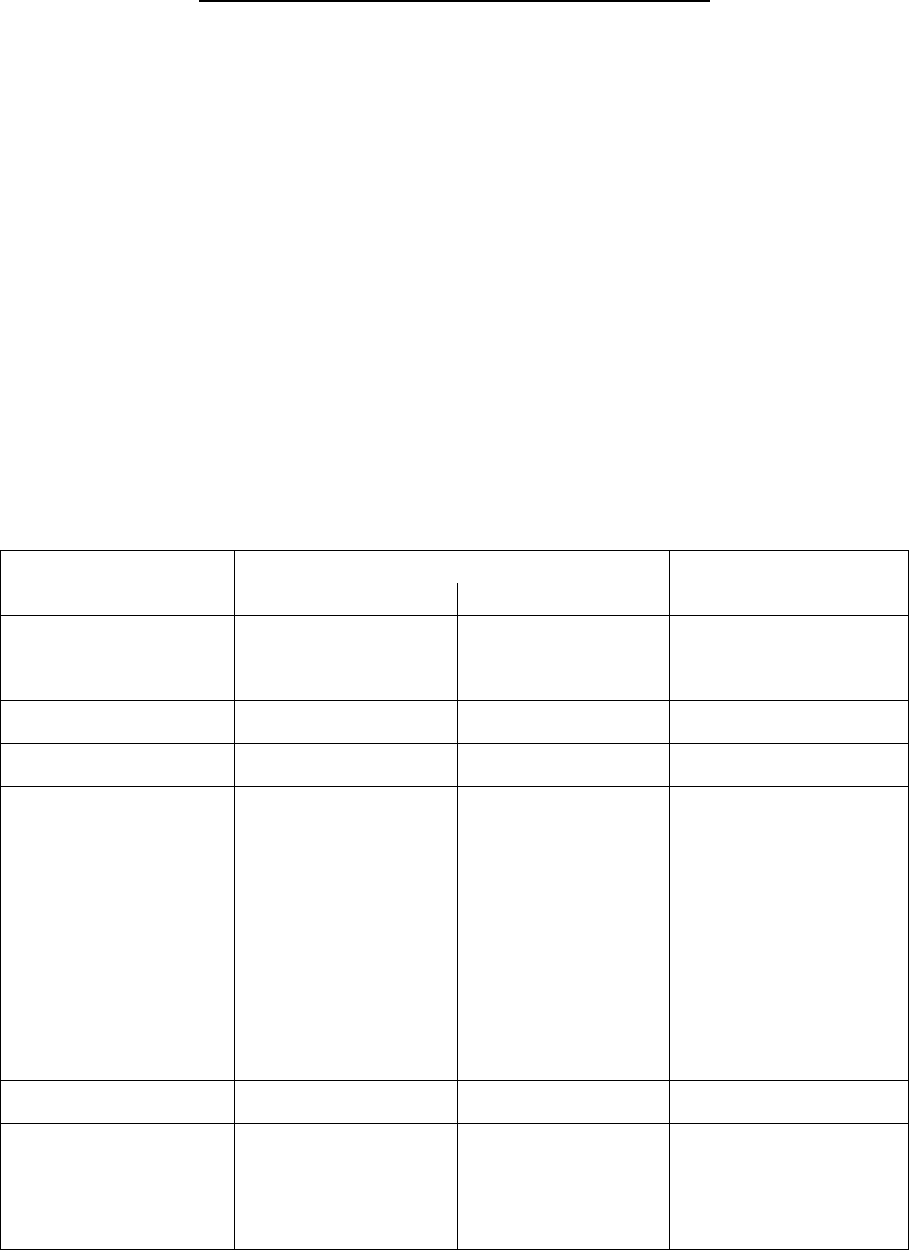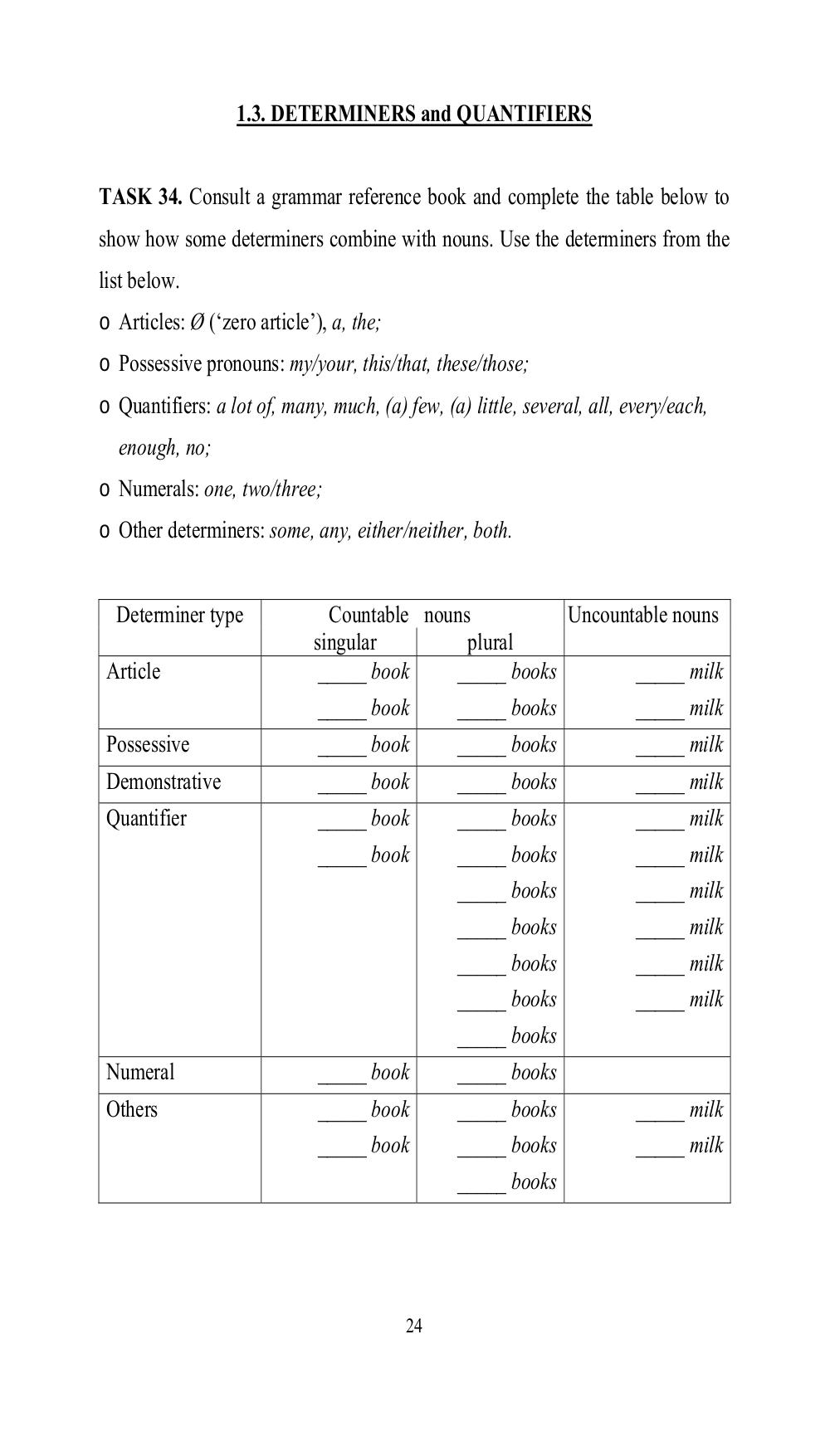# Focus on nominals: practice grammar book for intermediate learners of English. Бочарова М.В - 24 стр.

Составители:

Рубрика:

• ## Иностранный язык24
1.3. DETERMINERS and QUANTIFIERS
TASK 34. Consult a grammar reference book and complete the table below to
show how some determiners combine with nouns. Use the determiners from the
list below.
o Articles: Ø (‘zero article’), a, the;
o Possessive pronouns: my/your, this/that, these/those;
o Quantifiers: a lot of, many, much, (a) few, (a) little, several, all, every/each,
enough, no;
o Numerals: one, two/three;
o Other determiners: some, any, either/neither, both.
Determiner type Countable nouns Uncountable nouns
singular plural
Article
_____ book
_____ book
_____ books
_____ books
_____ milk
_____ milk
Possessive
_____ book _____ books _____ milk
Demonstrative
_____ book _____ books _____ milk
Quantifier
_____ book
_____ book
_____ books
_____ books
_____ books
_____ books
_____ books
_____ books
_____ books
_____ milk
_____ milk
_____ milk
_____ milk
_____ milk
_____ milk
Numeral
_____ book _____ books
Others
_____ book
_____ book
_____ books
_____ books
_____ books
_____ milk
_____ milk1.3. DETERMINERS and QUANTIFIERS

TASK 34. Consult a grammar reference book and complete the table below to
show how some determiners combine with nouns. Use the determiners from the
list below.
o Articles: Ø (zero article), a, the;
o Possessive pronouns: my/your, this/that, these/those;
o Quantifiers: a lot of, many, much, (a) few, (a) little, several, all, every/each,
enough, no;
o Numerals: one, two/three;
o Other determiners: some, any, either/neither, both.

Determiner type              Countable nouns          Uncountable nouns
singular         plural
Article                      _____ book    _____ books        _____ milk
_____ book    _____ books        _____ milk
Possessive                   _____ book        _____ books              _____ milk
Demonstrative                _____ book        _____ books              _____ milk
Quantifier                   _____ book        _____ books              _____ milk
_____ book        _____ books              _____ milk
_____ books              _____ milk
_____ books              _____ milk
_____ books              _____ milk
_____ books              _____ milk
_____ books
Numeral                      _____ book        _____ books
Others                       _____ book        _____ books              _____ milk
_____ book        _____ books              _____ milk
_____ books

24